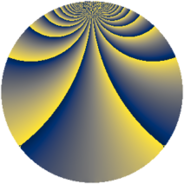Properties

 Label 588.1.zLevel $588$ Weight $1$ Character orbit 588.z Rep. character $\chi_{588}(53,\cdot)$ Character field $\Q(\zeta_{42})$ Dimension $12$ Newform subspaces $1$ Sturm bound $112$ Trace bound $0$

Related objects

Defining parameters

 Level: $$N$$ $$=$$ $$588 = 2^{2} \cdot 3 \cdot 7^{2}$$ Weight: $$k$$ $$=$$ $$1$$ Character orbit: $$[\chi]$$ $$=$$ 588.z (of order $$42$$ and degree $$12$$) Character conductor: $$\operatorname{cond}(\chi)$$ $$=$$ $$147$$ Character field: $$\Q(\zeta_{42})$$ Newform subspaces: $$1$$ Sturm bound: $$112$$ Trace bound: $$0$$

Dimensions

The following table gives the dimensions of various subspaces of $$M_{1}(588, [\chi])$$.

Total New Old
Modular forms 84 12 72
Cusp forms 12 12 0
Eisenstein series 72 0 72

The following table gives the dimensions of subspaces with specified projective image type.

$$D_n$$ $$A_4$$ $$S_4$$ $$A_5$$
Dimension 12 0 0 0

Trace form

 $$12 q + q^{3} + q^{7} + q^{9} + O(q^{10})$$ $$12 q + q^{3} + q^{7} + q^{9} + 2 q^{13} - q^{19} - 2 q^{21} + q^{25} - 2 q^{27} - q^{31} - 8 q^{37} - 8 q^{39} + 2 q^{43} + q^{49} + 2 q^{57} - 5 q^{61} - 6 q^{63} - q^{67} - q^{73} + q^{75} - q^{79} + q^{81} - q^{91} - q^{93} - 4 q^{97} + O(q^{100})$$

Decomposition of $$S_{1}^{\mathrm{new}}(588, [\chi])$$ into newform subspaces

Label Dim. $$A$$ Field Image CM RM Traces $q$-expansion
$a_{2}$ $a_{3}$ $a_{5}$ $a_{7}$
588.1.z.a $12$ $0.293$ $$\Q(\zeta_{21})$$ $D_{21}$ $$\Q(\sqrt{-3})$$ None $$0$$ $$1$$ $$0$$ $$1$$ $$q+\zeta_{42}^{10}q^{3}+\zeta_{42}^{8}q^{7}+\zeta_{42}^{20}q^{9}+\cdots$$